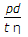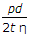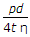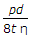# Mechanical Engineering - Strength of Materials

### Exercise :: Strength of Materials - Section 7

41.

The centre to centre distance, between two consecutive rivets in a row, is called

 A. margin B. pitch C. back pitch D. diagonal pitch

Explanation:

No answer description available for this question. Let us discuss.

42.

The buckling load for a given column depends upon

 A. area of cross-section of the column B. length and least radius of gyration of the column C. modulus of elasticity for the material of the column D. all of the above

Explanation:

No answer description available for this question. Let us discuss.

43.

A continuous beam is one which is

 A. fixed at both ends B. fixed at one end and free at the other end C. supported on more than two supports D. extending beyond the supports

Explanation:

No answer description available for this question. Let us discuss.

44.

When a body is subjected to two equal and opposite pulls, as a result of which the body tends to extend its length, the stress and strain induced is

 A. compressive stress, tensile strain B. tensile stress, compressive strain C. tensile stress, tensile strain D. compressive stress, compressive strain

Explanation:

No answer description available for this question. Let us discuss.

45.

The hoop stress in a riveted cylindrical shell of diameter (d), thickness (t) and subjected to an internal pressure (p) is (where η = Efficiency of the riveted joint)

 A.B.C.D.